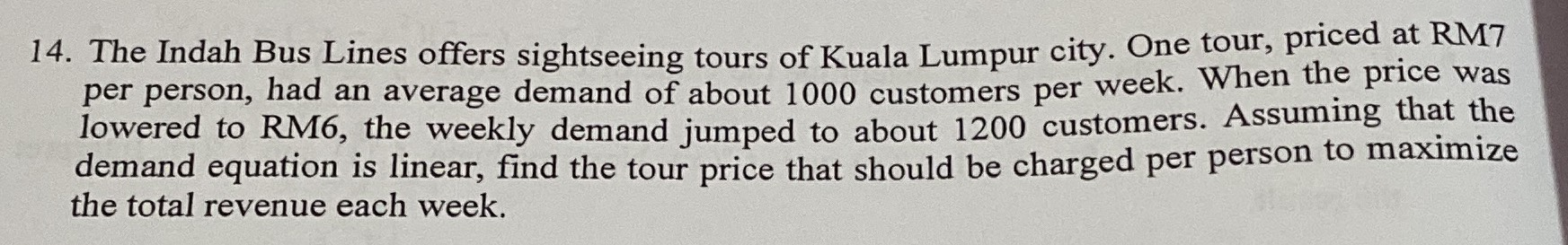### Still have math questions?

Algebra
QuestionThe Indah Bus Lines offers sightseeing tours of Kuala Lumpur city. One tour, priced at RM7 per person, had an average demand of about $$1000$$ customers per week. When the price was lowered to RM6, the weekly demand jumped to about $$1200$$ customers. Assuming that the demand equation is linear, find the tour price that should be charged per person to maximize the total revenue each week.

the rebenue is w=x[1000+200(7-x)]=-200x$$^{2}$$+2400x=-200(x$$^{2}$$-12x)=-200(x$$^{2}$$-12x+36-36)=-200(x-6)$$^{2}$$+7200